# SBI CLERK PRELIMS-REASONING DAY-13

Dear Bankersdaily Aspirants,

SBI Clerk Prelims 2018, will be going to held on March/April (Tentatively) 2018 as per SBI Clerk Notification. This Exam is going to be one of the toughest exam of the year 2018. Because SBI will be the game changer for all the upcoming bank exams. As per as the new pattern is concerned there will be sectional timing for three section in 1 hour. So as per the latest pattern each section is allotted with the sectional timing of 20 minutes.

NEW PATTERN:

Preliminary Examination:Phase I

Phase-I : Preliminary Examination: Preliminary Examination (online) consisting of Objective Tests for 100 marks will be conducted online. This test would be of 1 hour duration consisting of 3 Sections as follows:

<<>>

 SL. Name of test No. of Questions Marks Duration 1. English Language 30 30 20 Minutes 2. Numerical Ability 35 35 20 Minutes 3. Reasoning Ability 35 35 20 Minutes Total 100 100 1 Hour

So start your preparation now with this study Planner for SBI Clerk Prelims 2018. It’s a 30+ days complete planner with various Aptitude, Reasoning, English, topics Included.

Though the exam is getting tougher and tougher for each and every Year, Only consistently hard working candidates will crack the exam. So be a continuous hard worker and grab the opportunity to achieve your success.

SBI Clerk Prelims 2018 Study Planner Day-13

Reasoning-Mathematical Operations-3

Time: 20 Minutes

Can you take this Quiz in 20 minutes?

If you can then attend the quiz. Share your score in our Telegram Group.

You can join in our Telegram Channel the invite given below and you can share this with your friends and other aspirants who are preparing for their exams like you.

Caution: You should have Telegram app installed in your mobile or laptop to join the group. If you don’t have telegram app in your mobile. First install the app and click the link given below….

Click here to join Telegram Group

D.1-5):In each question, relationship between different elements is shown in the statements. The statements are followed by conclusions. Study the conclusions based on the given statements and select the appropriate answer.

Q.1) Statements:

M > O ≥ T ≤ I, S ≤ R ≥ H, I ≥ Q < R

Conclusions:

H ≤ M

Q < T

O > R

M < H

a) Only II follows

b) Only I and III follow

c) Only either I or IV follows

d) Only III and IV follows

e) None follows

Q.2) Statements:

L < I ≤ F, X> I > C ≥ R,  K >F >U<T

Conclusions:

R ≤ I

L < K

F > R

T > C

a) Only IV follows

b) Only II follows

c) Only III follows

d) Only I and II follow

e) Only II and III follows

Q.3) Statements:

B ≥ D ≥ F > H = J ≤ L < M = P

Conclusions:

M ≤ D

D > M

H > B

B ≥ H

a) None follows

b) Only either I or II follows

c) Only II and IV follows

d) Only either III or IV follows

e) Only I and III follow

Q.4) Statements:

L = S ≤ M, C ≥ T <E, A ≤ M < T

Conclusions:

C < A

S < E

A ≥ C

E > L

a) Only III follows

b) Only either I or III and II follows

c) Only either I or III follows

d) Only II and IV follows

e) None follows

Q.5) Statements:

R >O ≥F,     D <N ≤I,      U≥I >F

Conclusions:

R > D

U > O

D < U

F < N

a) Only I follow

b) Only II follows

c) Only III follows

d) Only IV follows

e) None of these

D.6-10): Study the following information carefully and answer the given questions

A@B means A is not smaller than B

A\$B means A is not greater than B

A%B means A is neither smaller than nor equal B

A#B means A is neither greater than nor equal B

A*B means A is neither greater than not smaller than B

Q.6) Statements:

L@M%P; H%G*P; S#N*O#H; K\$S.

Conclusion:

I.M#H

II. H%S

III. O%G

IV. G@N

a) Only II follow

b) Only III follow

c) Only I and II follow

d) Only I, II and IV follows

e) Only II and either III or IV

Q.7) Statement:

I*G@K%L*M\$N*O#P

Conclusion:

I.L%P

II. I@M

III. M#G

IV. O@L

a) Only II follow

b) Only III follow

c) Only I and II follow

d) Only III and IV follows

e) Only II and either III or IV

Q.8) Statements:

R%P; S@Q%O; V#T#R; W*V; P@U*S.

Conclusion:

I.Q\$P

II. R@W

III. U#T

IV. R%O

a) Only II follow

b) Only III and IV follows

c) Only I and III follow

d) Only I and IV follow

e) None of these

Q.9) Statement:

A@C%D*E\$F; B@H@G*D#I

Conclusion:

I.I#C

II. H%E

III. B@E

IV. I%E

a) Only II follow

b) Only III and IV follows

c) Only I and III follow

d) Only I and IV follow

e) None of these

Q.10) Statement:

P*R#Q\$T*U%W*X@Y

Conclusions:

I.P%T

II. T#Y

III. Y\$W

IV. U%P

a) Only II follow

b) Only II and IV follows

c) Only I and III follow

d) Only III and IV follows

e) None of these

Q.11) Which of the following symbols should replace the question mark in the given expression in order to make the expressions ‘Z ≤ V’ as well as ‘K > L’ definitely false?

Z≤L ? Q≤V<K≤T

a) ≤

b) =

c) ≥

d) Cannot be determined

e) None of these

12) Which of the following symbols should replace in the question mark, in the given expression so that the expressions ‘O > C’ and ‘B ≥ K’ definitely true?

O>Z≥K ?  C≤W=B

a) ≤

b) ≥

c) =

d) <

e) None of these

13) which of the following should be placed in the blank spaces in order to complete the given expression such that N < A is always true?
_ < _ ≤ _ >_

a) NLTA

b) NSTB

c) ANPC

d) NEAD

e) None of these

14) Which of the following symbol replace the question mark (?) in the given expression in order to make the expression L>N and P<M definitely true?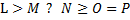a) ≤

b) ≥

c) <

d) >

e) None of these

15) which of the following groups of symbols should be placed in the blank space of the given expression to make T ≥ Q definitely false?

P_Q_R_S_T

a) <, ≤, ≤, =

b) >, ≥, =, >

c) >, =, ≤, ≤

d) ≤, =, =, ≤

e) None of these

D.16-20): In the following questions! ÷, +, – and × are used according to following Meanings:

‘A ! B’ means, ‘A is not smaller than B’

‘A ÷ B’ means, ‘A is not greater than B’

‘A + B’ means, ‘A is neither greater nor equal to B’

‘A – B’ means, ‘A is neither smaller nor greater than B’

‘A × B’ means, ‘A is neither smaller nor equal to B’

Now from the following statements, check whether the following conclusions I, II and III are definitely true.

Q.16) Statements:

B÷N, Q ! N, T×Q

Conclusions:

I.B+T

II.T×N

III. N ! B

a) None is true

b) Only I and II are true

c) Only III is true

d) All are true

e) Only II and III are true

Q.17) Statements:

K ! P, A×P, Q÷K

Conclusions:

I.Q÷A

II.K ! A

III. Q×P

a) Only I is true

b) Only II is true

c) Only III is true

d) None is true

e) All are true

Q.18) Statements:

X×Z, Z÷D, D-H

Conclusions:

I.H-Z

II.H+X

III. H × Z

a) All are true

b) Only either I or II are true

c) Only either I or III are true

d) Only either II or III are true

e) None of these

Q.19) Statements:

X-D, D!Y, Y+Z

Conclusions:

I.X-Z

II.X × Z

III. D × Z

a) Only I is true

b) None is true

c) Only III is true

d) Only II is true

e) All are true

Q.20) Statements:

U+R, R÷V, V-M

Conclusions:

I.M-R

II.M×R

III. M×U

a) All are true

b) Only either I or II are true

c) Only either I or II and III are true

d) Only either I or III and II are true

e) None of these

D.21-25): In these questions, a relationship between different elements is shown in the statement. Read the questions carefully and answer the following questions.

A@B means A is not smaller than B

A\$B means A is not greater than B

A%B means A is neither smaller than nor equal to B

A?B means A is neither greater than nor equal to B

A*B means A is neither greater than nor smaller than B

Q.21) If the conclusion F ? D and B % E is definitely true in which of the following statements that logically satisfies the given conclusion.

a) A ? B @D * F @ E ? C

b) D ? \$ * F % B @E * C

c) C % E * F % A \$ B ? D

d) D % C @ E * F ? A \$ B

e) None of these

Q.22) If the conclusion M \$ Q and R % O is definitely true in which of the following statements that logically satisfies the given conclusion.

a) L \$ M ? N \$ O * Q % R

b) R @ L * O % N * Q \$ M

c) O \$ N ? Q * R @ M % L

d) M @ O * L ? N * R % Q

e) None of these

Q.23) If the conclusion T \$ W and R % U is definitely true in which of the following statements that logically satisfies the given conclusion.

a) None of these

b) Q ? R * T ? U \$ W % X

c) X % R @ T * Q ?U ? W

d) Q % T * U @ X \$ R * W

e) W * R @ Q * T % U ? X

Q.24) If the conclusion either G * L or L % G is definitely true in which of the following statements that logically satisfies the given conclusion?

a) F % L @ E * K ? G * F

b) G \$ K * J \$ L % F @ E

c) E ? F \$ G * J @ K * L

d) K * L % J ? G * E ? F

e) None of these

Q.25) If the conclusion R % M and N \$ L are definitely false in which of the following statements that logically satisfies the given conclusion?

a) G * R ? L * M @ K * N

b) N @ K * M \$ L * G ? R

c) M * L @ G * N * R ? K

d) R @ G * L ? M * K \$ N

e) None of these

Explanation:

1.(c)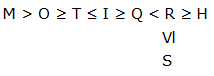H ≤ M (False)

Q < T (False)

O > R     (False)

M < H (False)

2.(e)

T > U < F ≥ I > C ≥ R;    L < I ≤ F < K

R ≤ I (False)

L < K (True)

F > R     (True)

T > C (False)

3.(a)

B ≥ D ≥ F > H = J ≤ L < M = P

M ≤ D (False)

D > M (False)

H > B     (False)

B ≥ H (False)

4.(d)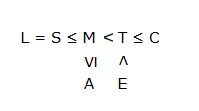C > A (False)

S < E (True)

A ≥ C     (False)

E > L (True)

5.(b)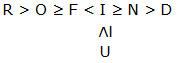R > D (False)

U > O (False)

D < U     (True)

F < N (False)

D.6-10):

@ –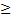\$ – ≤

% – >

# – <

* – =

6.(a)

L ≥ M > P;   H > G = P;   S < N = O < H;     K ≤ S

M < H (False)

H > S (True)

O > G    (False)

G ≥ N (False)

7.(d)

I = G ≥ K > L = M ≤ N = O < P

L > P (False)

I ≥ M (False)

M < G    (True)

O ≥ L (True)

8.(d)

R > P;          S ≥ Q > O;            V < T < R;   W= V;         P ≥ U = S

Q ≤ P (True)

R ≥ W (False)

U < T     (False)

R > O (True)

9.(b)

A ≥ C > D = E ≤ F; B ≥ H ≥ G = D < I

I < C (False)

H > E (False)

B ≥ E  (True)

I > E (True)

10.(d)

P = R < Q ≤ T = U > W = X ≥ Y

P > T (False)

T < Y (False)

Y ≤ W    (True)

U  P (True)

11.(c)

Z ≤ L ≥ Q ≤ V < K ≤ T

Z ≤ V (False)

K > L (False)

12.(c)

O > Z ≥ K = C ≤ W = B

O > C (True)

B ≥ K (True)

13.(d)

N < E ≤ A > D

So that N < A        (True)

14.(d)

L > M > N ≥ O = P

L > N (True)

P < M (True)

15.(b)

P > Q ≥ R = S > T

T ≥ Q           (False)

16.(e)

B ≤ N  Q  T

B < T (True)

T > N (True)

N ≥ B     (True)

17.(d)

Q ≤ K ≥ P < A

Q ≤ A (False)

K ≥ A (False)

Q > P     (False)

18.(c) Either I or III

X > Z ≤ D = H

H = Z (False)

H < X (False)

H > Z     (False)

19.(b)

X = D ≥ Y < Z

X = Z (False)

X > Z (False)

D > Z     (False)

20.(c)

U < R ≤ V = M

M = R (False)

M > R (False)

M > U    (True)

D.21-25):

@ – ≥

\$ – ≤

% – >

? – <

* – =

21.(d)

D > C ≥ E = F < A ≤ B

F < D (True)

B > E (True)

22.(c)

O ≤ N < Q = R ≥ M > L

M ≤ Q (True)

R > O (True)

23.(e)

W=R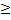Q=TUX

T ≤ W (True)

R > U (True)

24.(b)

G ≤ K = J ≤ L > F ≥ E

G = L, L > G

25.(d)

R ≥ G = L < M = K ≤ N

R > M (False)

N ≤ L (False)

You may also line: